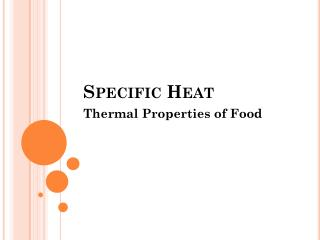DownloadDownload PresentationSpecific Heat

# Specific Heat

Download Presentation## Specific Heat

- - - - - - - - - - - - - - - - - - - - - - - - - - - E N D - - - - - - - - - - - - - - - - - - - - - - - - - - -
##### Presentation Transcript

1. Specific Heat Thermal Properties of Food

2. SPECIFIC HEAT Specific heat is the amount of heat required to increase the temperature of a unit mass of the substance by unit degree. Therefore, its unit is J/kg K in the SI system. The specific heat depends on the nature of the process of heat addition in terms of either a constant pressure process or a constant volume process.

3. However, because specific heats of solids and liquids do not depend on pressure much, except extremely high pressures, and because pressure changes in heat transfer problems of agricultural materials are usually small, the specific heat at constant pressure is considered (Mohsenin, 1980).

4. Prediction of Specific Heat The specific heats of foodstuffs depend very much on their composition. Knowing the specific heat of each component of a mixture is usually sufficient to predict the specific heat of the mixture (Sweat,1995). Heldman (1975) proposed the following equation to estimate the specific heat of foodstuffs using the mass fraction of its constituents (water, protein, fat, carbohydrate, and ash): cp= 4.180Xwwater + 1.547Xwprot + 1.672Xwfat + 1.42XwCHO + 0.836Xwash

5. Choi and Okos (1986) suggested the following equation for products containing n components: cp= ∑(Xwi . cpi ) Where: Xwi= mass fraction of component i , cpi= specific heat of component i (J/kg K).

6. The temperature dependence of specific heat of major food components has been studied. The specific heat of pure water, carbohydrate (CHO), protein, fat, ash, and ice at different temperatures can be expressed empirically in J/kg◦C according to Choi and Okos (1986) as follows: cpwater = 4081.7 − 5.3062 T + 0.99516T 2 (for −40 to 0◦C) cpwater = 4176.2 − 0.0909 T + 5.4731 × 10−3T 2 (for 0 to 150◦C) cpCHO = 1548.8 + 1.9625 T − 5.9399 × 10−3T 2 (for −40 to 50◦C)

7. cpprot = 2008.2 + 1.2089 T − 1.3129 × 10−3T 2 (for−40to150◦C) cpfat = 1984.2 + 1.4373 T − 4.8008 × 10−3T2 (for −40 to 150◦C) cpash = 1092.6 + 1.8896 T − 3.6817 × 10−3T 2 (for −40 to 150◦C) cpice = 2062.3 + 6.0769 T where temperature (T ) is in (◦C) in these equations.

8. Specific heat of moist air can be expressed as a function of relative humidity (RH) of air (Riegel,1992): cpmoist air = cpdry air(1 + 0.837RH)

9. Generally, experimentally determined specific heat is higher than the predicted value. The reason may be the presence of bound water, variation of specific heat of the component phases with the source and interaction of the component phases (Rahman, 1995). Rahman (1993) considered the excess specific heat, cex, due to the interaction of the component phases and proposed the following equation:

10. These equations are valid in a temperature change when there is no phase change. If there is a phase change, latent heat must be incorporated. This is accomplished by using a new term, apparent specific heat, which includes both latent and sensible heat:

11. Estimate the specific heat of potatoes containing 85% water. Data: cpwater= 4186.80 J/kgK cpnonfat solids = 837.36 J/kgK

12. Solution: Using Eq. (3.95) as suggested by Choi and Okos (1986):

13. Approximate Composition of Wild Rice Component Weight (%) Water 8.5 Carbohydrate 75.3 Protein 14.1 Fat 0.7 Ash 1.4 Determine the specific heat of wild rice grain at 20°C using Heldman’s equation and Choi and Okos’s equations. Compare results.